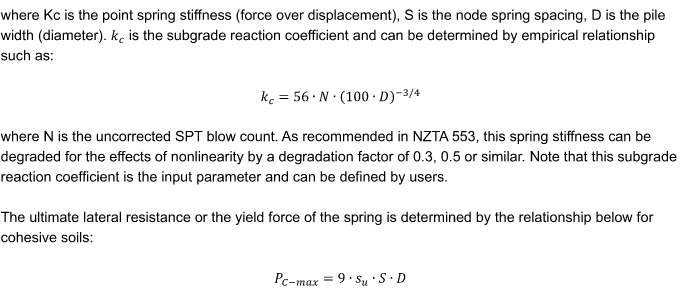top of page

# P-y curves for liquefaction and lateral spreading effects based on NZTA 553

Pseudo-static analysis for pile foundations subject to liquefaction and lateral spreading requires the using of appropriate p-y curve models. NZ Transport Agency research report 553 - the development of design guidance for bridges in New Zealand for liquefaction and lateral spreading effects published in 2014 recommends three different bilinear elastic-plastic p-y curves for soils which can be used for analysis of piles undergoing lateral spreading in liquefied soils.As shown in the figure above (extracted from NZTA 553), three p-y curve models are for (1) non-liquefied crust layer, (2) liquefied soil layer and (3) non-liquefied base layer.

Note that the identical procedure is adopted for the non-liquefied crust layer and non-liquefied base layer. The only difference is the different empirical parameter (Alpha - strength scaling factor) used to estimate the ultimate lateral resistance for cohesionless soils. As a result of that, the following p-y curve models are included in the PileLAT program:

1. Non-liquefied crust for cohesive soils

2. Non-liquefied cohesionless soils

3. Liquefied soils

1. Non-liquefied crust for cohesive soils

The p-y curve of non-liquefied crust for cohesive soils as recommended in NATA 553 is shown in the figure below.The soil spring stiffness is defined in the relationship below:The required input parameters for non-liquefied crust for cohesive soils (NZTA 553) in PileLAT are shown in the figure below and summarized as follows:

• Total Unit Weight: The total unit weight of the soil material

• Undrained Shear Strength: Undrained shear strength at the top of the soil layer with the input material set.

• Su – Rate of Change: Increase rate of the undrained shear strength from the top of the soil layer with the input material set.

• Subgrade Reaction Coefficient, kc: Subgrade reaction coefficient at the top pf the soil layer with the input material set.

• kc, Rate of Change: Increase rate of subgrade reaction coefficient from the top of the soil layer with the input material set.2. Non-liquefied cohesionless soils

The p-y curve model for non-liquefied cohesionless soils covers two different p-y curve relationships recommended in NZTA 553 for (1) non-liquefied crust for cohesionless soils and (2) non-liquefied deeper soils.

The p-y curve shape is identical to the above figure. The spring stiffness determination is the same as the non-liquefied crust for cohesive soils (NZTA 553). The only difference is the estimation of the ultimate lateral resistance, or the yield force of the spring based on the equation below:The required input parameters for non-liquefied cohesionless soils (NZTA 553) in PileLAT are shown in the figure below and summarized as follows:

• Total Unit Weight: The total unit weight of the soil material

• Effective Friction Angle: Effective friction angle of the soil material.

• Scaling Factor, Alpha-c: Strength scaling factor for cohesionless soils.

• Subgrade Reaction Coefficient, kc: Subgrade reaction coefficient at the top pf the soil layer with the input material set.

• Kc, Rate of Change: Increase rate of subgrade reaction coefficient from the top of the soil layer with the input material set.3. Non-liquefied crust for cohesive soils

The p-y curve of liquefied soils as recommended in NATA 553 is shown in the figure below.The soil spring stiffness is defined in the relationship below:The required input parameters for liquefied soils (NZTA 553) in PileLAT are shown in the figure below and summarized as follows:

• Total Unit Weight: The total unit weight of the soil material

• Residual Strength, Sr: Residual strength at the top of the soil layer with the input material set.

• Sr – Rate of Change: Increase rate of the residual strength from the top of the soil layer with the input material set.

• Subgrade Reaction Coefficient, kL: Subgrade reaction coefficient at the top pf the soil layer with the input material set.

• kL, Rate of Change: Increase rate of subgrade reaction coefficient from the top of the soil layer with the input material set.

• Scaling Factor, Alpha-L: Strength scaling factor for liquefied soils.